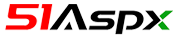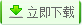| | 帮助?

[免费版 Free] WebForm,下载次数：349 次 | 关键字： 考试 办公 高校 竞赛 企事业单位## 源码目录树

;
```当前路径：vendor/phpunit/phpunit/tests/unit/Framework/Constraint/IsEqualTest.php
<?php declare(strict_types=1);
/*
* This file is part of PHPUnit.
*
* (c) Sebastian Bergmann <sebastian@phpunit.de>
*
* file that was distributed with this source code.
*/
namespace PHPUnit\Framework\Constraint;

use PHPUnit\Framework\ExpectationFailedException;
use PHPUnit\Framework\TestFailure;

/**
* @small
*/
final class IsEqualTest extends ConstraintTestCase
{
public function testConstraintIsEqual(): void
{
\$constraint = new IsEqual(1);

\$this->assertTrue(\$constraint->evaluate(1, '', true));
\$this->assertFalse(\$constraint->evaluate(0, '', true));
\$this->assertEquals('is equal to 1', \$constraint->toString());
\$this->assertCount(1, \$constraint);

try {
\$constraint->evaluate(0);
} catch (ExpectationFailedException \$e) {
\$this->assertEquals(
<<<EOF
Failed asserting that 0 matches expected 1.

EOF
,
TestFailure::exceptionToString(\$e)
);

return;
}

\$this->fail();
}

/**
* @dataProvider isEqualProvider
*/
public function testConstraintIsEqual2(\$expected, \$actual, \$message): void
{
\$constraint = new IsEqual(\$expected);

try {
\$constraint->evaluate(\$actual, 'custom message');
} catch (ExpectationFailedException \$e) {
\$this->assertEquals(
"custom message\n\$message",
\$this->trimnl(TestFailure::exceptionToString(\$e))
);

return;
}

\$this->fail();
}

public function testConstraintDeltaIsNotZero(): void
{
\$constraint = new IsEqual(15, 1);

\$this->assertSame('is equal to 15 with delta <1.000000>', \$constraint->toString());
}

public function isEqualProvider(): array
{
\$a      = new \stdClass;
\$a->foo = 'bar';
\$b      = new \stdClass;
\$ahash  = \spl_object_hash(\$a);
\$bhash  = \spl_object_hash(\$b);

\$c               = new \stdClass;
\$c->foo          = 'bar';
\$c->int          = 1;
\$c->array        = [0, , , 3];
\$c->related      = new \stdClass;
\$c->related->foo = "a\nb\nc\nd\ne\nf\ng\nh\ni\nj\nk";
\$c->self         = \$c;
\$c->c            = \$c;
\$d               = new \stdClass;
\$d->foo          = 'bar';
\$d->int          = 2;
\$d->array        = [0, , , 3];
\$d->related      = new \stdClass;
\$d->related->foo = "a\np\nc\nd\ne\nf\ng\nh\ni\nw\nk";
\$d->self         = \$d;
\$d->c            = \$c;

\$storage1 = new \SplObjectStorage;
\$storage1->attach(\$a);
\$storage1->attach(\$b);
\$storage2 = new \SplObjectStorage;
\$storage2->attach(\$b);
\$storage1hash = \spl_object_hash(\$storage1);
\$storage2hash = \spl_object_hash(\$storage2);

\$dom1                     = new \DOMDocument;
\$dom1->preserveWhiteSpace = false;
\$dom2                     = new \DOMDocument;
\$dom2->preserveWhiteSpace = false;

return [
[1, 0, <<<EOF
Failed asserting that 0 matches expected 1.

EOF
],
[1.1, 0, <<<EOF
Failed asserting that 0 matches expected 1.1.

EOF
],
['a', 'b', <<<EOF
Failed asserting that two strings are equal.
--- Expected
+++ Actual
@@ @@
-'a'
+'b'

EOF
],
["a\nb\nc\nd\ne\nf\ng\nh\ni\nj\nk", "a\np\nc\nd\ne\nf\ng\nh\ni\nw\nk", <<<EOF
Failed asserting that two strings are equal.
--- Expected
+++ Actual
@@ @@
'a\\n
-b\\n
+p\\n
c\\n
d\\n
e\\n
@@ @@
g\\n
h\\n
i\\n
-j\\n
+w\\n
k'

EOF
],
[1, , <<<EOF
Array (...) does not match expected type "integer".

EOF
],
[, 1, <<<EOF
1 does not match expected type "array".

EOF
],
[, , <<<EOF
Failed asserting that two arrays are equal.
--- Expected
+++ Actual
@@ @@
Array (
-    0 => 0
+    0 => 1
)

EOF
],
[[true], ['true'], <<<EOF
Failed asserting that two arrays are equal.
--- Expected
+++ Actual
@@ @@
Array (
-    0 => true
+    0 => 'true'
)

EOF
],
[[0, , , 3], [0, , , 3], <<<EOF
Failed asserting that two arrays are equal.
--- Expected
+++ Actual
@@ @@
Array (
0 => 0
1 => Array (
-        0 => 1
+        0 => 4
)
2 => Array (...)
3 => 3
)

EOF
],
[\$a, , <<<EOF
Array (...) does not match expected type "object".

EOF
],
[, \$a, <<<EOF
stdClass Object (...) does not match expected type "array".

EOF
],
[\$a, \$b, <<<EOF
Failed asserting that two objects are equal.
--- Expected
+++ Actual
@@ @@
stdClass Object (
-    'foo' => 'bar'
)

EOF
],
[\$c, \$d, <<<EOF
Failed asserting that two objects are equal.
--- Expected
+++ Actual
@@ @@
stdClass Object (
'foo' => 'bar'
-    'int' => 1
+    'int' => 2
'array' => Array (
0 => 0
1 => Array (
-            0 => 1
+            0 => 4
)
2 => Array (...)
3 => 3
@@ @@
)
'related' => stdClass Object (
'foo' => 'a\\n
-        b\\n
+        p\\n
c\\n
d\\n
e\\n
@@ @@
g\\n
h\\n
i\\n
-        j\\n
+        w\\n
k'
)
'self' => stdClass Object (...)
'c' => stdClass Object (...)
)

EOF
],
[\$dom1, \$dom2, <<<EOF
Failed asserting that two DOM documents are equal.
--- Expected
+++ Actual
@@ @@
<?xml version="1.0"?>
-<root/>
+<root>
+  <foo/>
+</root>

EOF
],
[
new \DateTime('2013-03-29 04:13:35', new \DateTimeZone('America/New_York')),
new \DateTime('2013-03-29 04:13:35', new \DateTimeZone('America/Chicago')),
<<<EOF
Failed asserting that two DateTime objects are equal.
--- Expected
+++ Actual
@@ @@
-2013-03-29T04:13:35.000000-0400
+2013-03-29T04:13:35.000000-0500

EOF
],
[\$storage1, \$storage2, <<<EOF
Failed asserting that two objects are equal.
--- Expected
+++ Actual
@@ @@
-SplObjectStorage Object &\$storage1hash (
-    '\$ahash' => Array &0 (
-        'obj' => stdClass Object &\$ahash (
-            'foo' => 'bar'
-        )
-        'inf' => null
-    )
-    '\$bhash' => Array &1 (
+SplObjectStorage Object &\$storage2hash (
+    '\$bhash' => Array &0 (
'obj' => stdClass Object &\$bhash ()
'inf' => null
)
)

EOF
],
];
}

/**
* Removes spaces in front of newlines
*
* @param string \$string
*
* @return string
*/
private function trimnl(\$string)
{
return \preg_replace('/[ ]*\n/', "\n", \$string);
}
}
```

Floor 5th,Daheng Building,Zhongguancun,Beijing,China,100080